# Creator Tree-Geometries

Geometry sections are used to create the environment in which your Bulk Material acts: for example, a pipe or a mixing drum. Sections can be defined in EDEM or imported from a CAD (or similar) package. Particle factories are also created using sections.

A model can have any number of sections allowing complex machinery to be created. A section can be defined as a box, a cylinder or as an n-sided polygon. Each section comprises a number of triangular elements: For example a square surface is made up of two elements and a ten-sided polygon of ten elements.

To create a new section :

1. Right click on the Geometries section of the Creator Tree

2. Choose Right Click > Add Geometry and Choose Box, Cylinder or Polygon. You can also use the Icon System.

3. Right Click the selected Geometry to rename as appropriate.

Select the Geometry to see the General Properties:

 Properties Description Volume A section is described as a volume or a surface. Completely closed boxes and cylinders are volumes; polygons and open boxes or cylinders are surfaces. This field is completed automatically. Volumes or virtual surfaces can later be turned into particle factories. Material Select a Geometry material from the drop-down list. Type A section is either Virtual, Physical or Dynamic Domain. A physical section is an actual surface or volume that particles can interact with. A virtual section (used to create particle factories) is a surface or volume of interest that does not actually exist and does not interact with anything in a simulation. A Dynamic Domain can only be applied to an EDEM Box geometry (volume). If the Simulator Dynamic Domain option is checked then any particles inside the Dynamic Domain will be active and particle contacts and forces are calculated as usual. Any particles outside the dynamic domain will be assessed to determine if they are stationary. Any stationary particles will be frozen and removed from the contact detection calculations, allowing the simulation to be resolved faster. Parent Allows users to define a Geometry object to be a parent if using the parent child functionality.

Expand the section in the Creator Tree to see the Mass, Electrostatics (if appropriate), Transform  and Section Properties:

 Property Description Mass EDEM automatically calculates the center of mass of the Geometry.  If required, set the center of mass parameters for X, Y, and Z components (for imported Geometries) or click the calculate option to adjust the Center of Mass to the Geometries current position. For open volumes the CoM is calculated based on the size and center of the mesh elements. The mass is automatically calculated based on the density of the equipment material.  If auto-calculate is not ticked this value can be modified. The Moment of inertia is based on the shape of the equipment.  It can be manually defined by unticking the auto calculation option. Electrostatics Set the Electrostatic charge of the material. (Electrostatics is hidden by default) Box Center. Set the position of the center of the box. Dimensions. Set the box length in the X, Y and Z axis. Enable Faces. Any of the six sides of the box can be removed. Cylinder Radius Start. Set the radius of one end of the cylinder. The end can be open or closed. Radius End. Sets the radius of one end of the cylinder. The end can be open or closed. Length. Set the distance between the start and end radius. Polygon All polygons, except a four-sided polygon are defined as follows: Edges. Set the number of edges. The minimum number is three. Radius. Set the size of the radius from the center to the corner of the shape A four-sided polygon is specified as follows: Edges. Set the number of edges to four. Dimensions. Set the length and the width. Transform See Section on Transform below

Note: Geometry sections outside of or directly coincident with the domain boundary do not take part in the simulation, and will hence be 'invisible' to particles.

### Transform

The transform menu allows you to define the position and orientation of your Geometry.  The menu is the same for imported CAD parts, boxes, cylinders and polygons. Transforms can be applied globally or locally.

 Position Set the X, Y and Z position of the object Rotation Set the X, Y and Z rotation of the object this is relative to the origin of the object.  To move the point of rotation, use the Local Origin Offset (see below) Note: Rotation transforms can only be defined at the first time step. Local Axis Rotation Rotations can be made using these buttons. The > will rotate the object by 1 degree and >> will rotate the object by 5 degrees Local Origin Offset The rotation acts relative to the local origin. The local origin is automatically set to the Geometries center of mass

#### Alternative Rotation Option

It is also possible to use the secondary rotation option.  This method rotates an object about a Vector.

 Start Point Enter the X Y and Z coordinate of the start point of the vector Alternatively: Use the Pick button to select aGeometry element as a starting point End point. Enter the X Y and Z coordinate of the end point of the vector Alternatively: Use the Pick button to select a Geometry element as an ending point Angle Specify the angle to rotate about the vector Apply This will update the parameters defined in the Transform section

NB. The axis key for the transform can be turned On or Off by going to View>Geometry Origin Axes and ticking or unticking the option.

### Example Geometry Sections Parts

Closed Box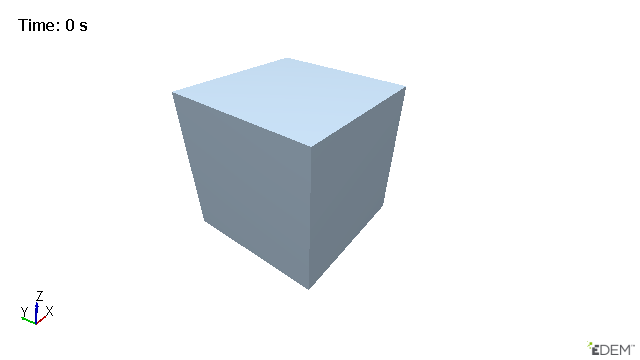Length (m) Position (m) Rotation (rad) X 0.5 0.5 0 Y 0.5 0.5 0 Z 0.5 0.5 0

Open Tilted Cuboid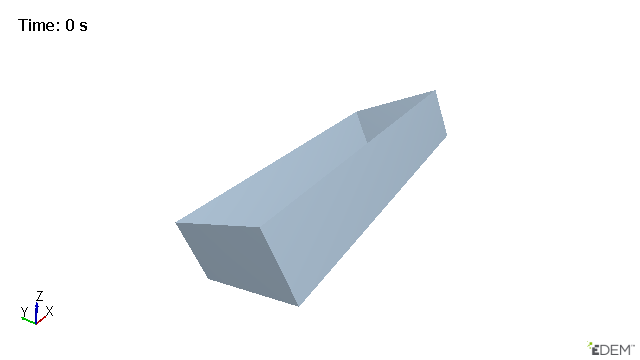Length (m) Position (m) Rotation (rad) X 0.5 0.5 -0.5 Y 0.2 0.5 0 Z 0.1 0.5 0

Vertical Cylinder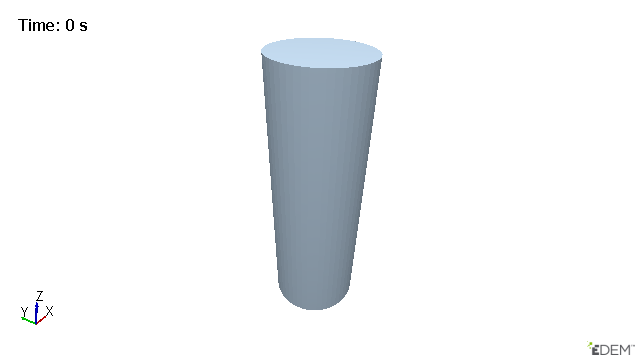Position (m) Rotation (rad) Radius Start (m) 0.1 X 0.5 -0.5 Radius End (m) 0.1 Y 0.5 0 Length (m) 0.6 Z 0.5 0

Cylinder Tilted in the y Direction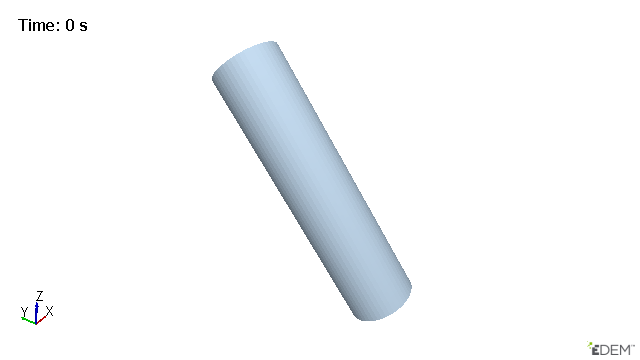Position (m) Rotation (rad) Radius Start (m) 0.1 X 0.5 -0.7 Radius End (m) 0.1 Y 0.75 0 Length (m) 0.78 Z 0.5 0

Funnel Tilted in the x Direction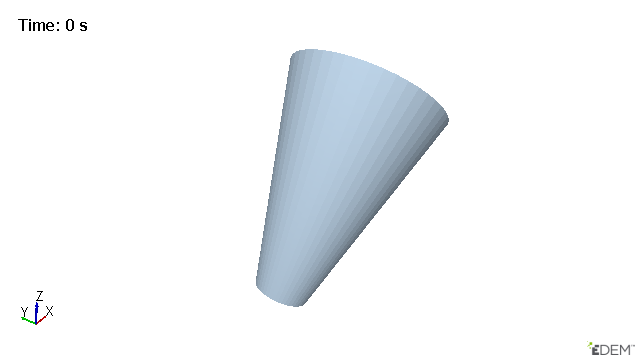Position (m) Rotation (rad) Radius Start (m) 0.1 X 0.7 0 Radius End (m) 0.3 Y 0.5 0.7 Length (m) 0.78 Z 0.5 0

6 Sided Polygon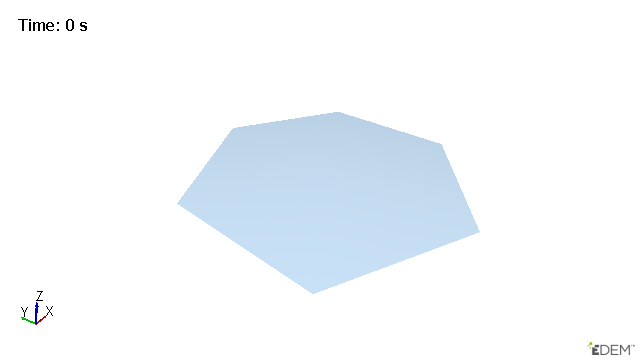Position (m) Rotation (rad) Radius X 0.7 0 0.5 Y 0.5 0 Z 0.5 0

4 Sided Tilted Polygon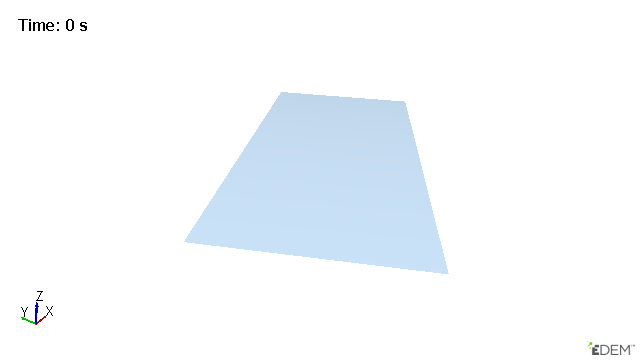Length A Position (m) Rotation (rad) 1 X 0.5 0 Length B Y 0.5 0 1 Z 0.2 0.5

3 Sided Polygon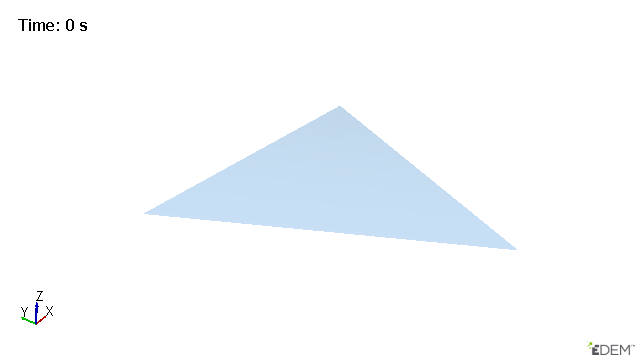Position (m) Rotation (rad) Radius X 0.5 0 0.5 Y 0.5 0 Z 0.3 0

### Parent Child Functionality

Geometry handling in EDEMnow enables Geometry sections to be defined as ‘children’ of another geometry section. This allows the ‘child’ geometry to inherit kinematics from the ‘parent’ geometry which greatly reduces the number of kinematics which need to be created in complex systems. At this point the primary benefit of this change is the ability for the Dynamic Domain to be defined as a child of another geometry section without the need for movement to be controlled by an external 3rd party such as MBD software.

Once the hierarchy has been defined in the tree it can be identified by the indent relative to the parent Geometry as can be seen below: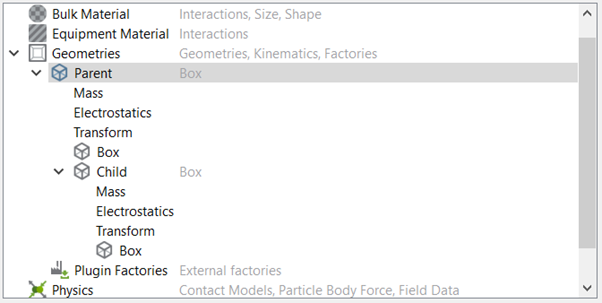The child will now move with the parent if the parent is assigned a motion or is moved within global space (rotated or translated).

Before setting the initial position of the parent geometry, it is best practice to assign the child and select or deselect the “Include Rotation From Parent Transform" check-box (located within the child's Transform). This will mitigate any unwanted movements when selecting or deselecting “Include Rotation From Parent Transform".

#### Partial Parent Transform

There is the option located within the Child’s Transform to not include the rotational aspect of the parent’s motion. This can be found in Geometry > “Parent Geometry” > “Child Geometry” > Transform > Partial Parent Transform. Deselect this option to only retain the parent translational motions.

### Specify Geometry Motions

Sections of geometry can be static or dynamic. Static sections remain in a fixed position during the course of a simulation whereas dynamic sections move. Sections can move under fixed translation, rotation, conveyor motion or sinusoidal motion.

#### Specifying a Kinematic

When kinematics are used to define the equipment they will not move when a force provided by another element is applied. For example, a section will not move when another section comes into contact with it or when a particle bounces off it. Sections pass through each other when they come into contact.

#### Specifying a Translation

1. Select the required geometry in the Creator Tree > Geometries section.

2. Right Click > Add Motion > Choose Linear Kinematic or Sinusoidal Translation Kinematic (used to model oscillating motion). Alternatively use the icon system.

3. Rename the kinematic as appropriate.

 Translation Setup Linear Set the Start time and End time. This determines the points in the simulation at which the motion will begin and end. The Loop Every tick box enables the kinematic to be looped, for example a kinematic with start time of Xs and end time of Ys with Loop Every set to Zs would run from Xs to Ys then repeat again at (X+Z)s to (Y+Z)s and again at (X+2Z)s to (Y+2Z)s until the Until Time is reached. Set the Initial velocity of the section. This determines the speed of the section at the specified start time. Specify the Acceleration of the section. Acceleration can be set to 0. Set the direction vector for the motion. This determines the direction the section will move in. Sinusoidal Set the Start time and End time. This determines the points in the simulation at which the motion will begin and end. The Loop Every tick box enables the kinematic to be looped, for example a kinematic with start time of Xs and end time of Ys with Loop Every set to Zs would run from Xs to Ys then repeat again at (X+Z)s to (Y+Z)s and again at (X+2Z)s to (Y+2Z)s until the Until Time is reached. Specify the Frequency of the oscillation. Specify the oscillation Offset: for example, two sections with identical oscillations offset by 180° will travel in opposite directions. Set the Displacement Magnitude (also known as the amplitude) of the oscillation in each direction.

#### Specifying a Rotation

• Select the required geometry in the Creator Tree > Geometries section.

• Right Click > Add Motion > Choose Linear Kinematic or Sinusoidal Rotation Kinematic (used to model oscillating motion). Alternatively use the icon system.

• Rename the kinematic as appropriate.

 Translation Setup Linear Set the Start time and End time. This determines the points in the simulation at which the rotation will begin and end. The Loop Every tick box enables the kinematic to be looped, for example a kinematic with start time of Xs and end time of Ys with Loop Every set to Zs would run from Xs to Ys then repeat again at (X+Z)s to (Y+Z)s and again at (X+2Z)s to (Y+2Z)s until the Until Time is reached. Set the Initial velocity of the section. This determines how fast the section will start rotating at the specified start time. Specify the Acceleration of the section. Acceleration can be set to 0. Set the axis of rotation. This defines around which axis the section will rotate. Specify the point of action (the center of the rotation). If the geometry section is also moving under a translation, select the Moves With Body option to update center as the section moves. This will ensure that the center of the rotation remains in the same relative position to the geometry section. Sinusoidal Set the Start time and End time. This determines the points in the simulation at which the motion will begin and end. The Loop Every tick box enables the kinematic to be looped, for example a kinematic with start time of Xs and end time of Ys with Loop Every set to Zs would run from Xs to Ys then repeat again at (X+Z)s to (Y+Z)s and again at (X+2Z)s to (Y+2Z)s until the Until Time is reached. Specify the Frequency of the oscillation.  Specify the oscillation Offset. Set the Displacement Magnitude (angle) of the oscillation in each direction. Specify the point of action (the center of the rotation). If the geometry section is also moving under a translation, select the Moves with body option to update center as the section moves. This will ensure that the center of the rotation remains in the same relative position to the geometry section.

#### Specifying a Conveyor

• Select the required geometry in the Creator Tree > Geometries section.

• Right Click > Add Motion > Choose Conveyor Translation or Conveyor Rotation.

• Rename the Motion as appropriate.

The conveyor motion will add a relative motion to the equipment section. Unlike the Linear Translation or Linear Rotation that equipment section will not move, the particles will experience the relative velocity of the conveyor belt like motion.

 Conveyor Setup Translation Set the Start time and End time. This determines the points in the simulation at which the motion will begin and end.  The Loop Every tick box enables the kinematic to be looped, for example a kinematic with start time of Xs and end time of Ys with Loop Every set to Zs would run from Xs to Ys then repeat again at (X+Z)s to (Y+Z)s and again at (X+2Z)s to (Y+2Z)s until the Until Time is reached.  Set the Initial velocity of the section. This determines the speed of the section at the specified start time. Specify the Acceleration of the section. Acceleration can be set to 0. Set the direction vector for the motion. This determines the direction the section will move in. Rotation Set the Start time and End time. This determines the points in the simulation at which the motion will begin and end. The Loop Every tick box enables the kinematic to be looped, for example a kinematic with start time of Xs and end time of Ys with Loop Every set to Zs would run from Xs to Ys then repeat again at (X+Z)s to (Y+Z)s and again at (X+2Z)s to (Y+2Z)s until the Until Time is reached.   Set the Initial velocity of the section. This determines how fast the section will start rotating at the specified start time. Specify the Acceleration of the section. Acceleration can be set to 0. Set the axis of rotation. This defines around which axis the section will rotate. Specify the point of action (the center of the rotation). If the geometry section is also moving under a translation, select the Moves With Body option to update center as the section moves. This will ensure that the center of the rotation remains in the same relative position to the geometry section.

Note: When setting a series of kinematics to a single geometry the order that the kinematics appear in the creator tree is important, particularly when the point of action is moving with the body. Kinematics should be arranged in the following order: 1) Translational Kinematics, 2) Rotational Kinematics, 3) Conveyor Kinematics. You can move kinematics up or down in the creator tree by right clicking on the kinematic and selecting “Move Up” and “Move Down”.

#### Specifying a Motion Controller

Unlike kinematics, motion controllers can be added to the geometry elements to allow objects to react to particle-geometry forces.  Forces acting on the geometry are included in the calculation of the geometries motion.  There is no contact detection between geometry elements.  Sections pass through each other when they come into contact.

Motion control uses the Geometry Mass to calculate accelerations, the mass is automatically calculated in the Geometries Tree and can be manually set by the user. This should be representative of the real geometry mass and a non-zero value.

NB. Particle-geometry interactions between particles outside the dynamic domain and geometries with force and torque motions are not supported.  This is due to the particle-geometry forces not being calculated in these instances.

#### Specifying a Force

1. Select the required geometry in the Creator Tree > Geometries section.
2. Right Click > Add Motion > Add Force Controller. Alternatively use the icon system.
3. Rename the Motion as appropriate.
 Force Controller Set the Start time and End time. This determines the points in the simulation at which the motion will begin and end.  The Loop Every tick box enables the motion to be looped, for example a kinematic with start time of Xs and end time of Ys with Loop Every set to Zs would run from Xs to Ys then repeat again at (X+Z)s to (Y+Z)s and again at (X+2Z)s to (Y+2Z)s until the Until Time is reached.  Set an equation for the force. This can be a single value or an equation. Set the Initial force of the section. This determines the initial force acting on the section at the specified start time. Tick the gravity option to add a gravity force to the object.  This is based on the mass of the section (and can be changed in the Mass section of the Section) Add a Damping coefficient.  Damping is calculated by CmV where C - Damping coefficient m - Mass of the object and V is the velocity of the object You can constrain the motion in 1 or 2 dimensions.  These dimensions are defined as a vector in the X, Y and Z direction. The motion can be constrained to a maximum speed.  This can be done by ticking the “enable speed cap option” and inputting a maximum speed value.

#### Specifying a Torque

 Torque Controller Set the Start time and End time. This determines the points in the simulation at which the motion will begin and end.  The Loop Every tick box enables the motion to be looped, for example a kinematic with start time of Xs and end time of Ys with Loop Every set to Zs would run from Xs to Ys then repeat again at (X+Z)s to (Y+Z)s and again at (X+2Z)s to (Y+2Z)s until the Until Time is reached.  Set an equation for the torque. This can be a single value or an equation. Set the Initial torque on the section. This determines the initial torque acting on the section at the specified start time. Tick the gravity option to add a gravity force to the object.  This is based on the mass of the section (and can be changed in the Mass section of the Section) Add a Damping Coefficient.  Damping is calculated by CωI where C - Damping coefficient, I - Moment of Inertia of the object and ω is the angular velocity of the object. You can constrain the motion in 1 dimension this axis is defined by a vector defined by X, Y and Z components.  The amount of displacement can be capped in both directions, this is done by ticking the box and inputting high and low values for the Angular displacements The motion can be constrained to a maximum angular speed.  This can be done by ticking the “enable speed cap option” and inputting a maximum speed value. Specify the point of action (the center of the rotation). If the geometry section is rotating about the start point of the torque controller select the "Start point" option.  To define a specific point use the "Manual Point" option.  If the torque applies about the Center of Mass of the object select the "Use Center of Mass Option. If the geometry section is also moving under a translation, select the Moves with body option to update center as the section moves. This will ensure that the center of the rotation remains in the same relative position to the geometry section.

#### Force / Torque Inputs as Equations

The input fields for both torque and force can take equations, as well as numerical values. These fields take equations in time, parameterized as t, which can be used to create a wide-range of behavior. No other parameters are currently accepted, so you must write your equations in terms of t, and not x, y ,z,  nor any other.

A list of the math functions which do work is here:

 Method Description abs(x) Returns the absolute value of x acos(x) Returns the arccosine of x, in radians asin(x) Returns the arcsine of x, in radians atan(x) Returns the arctangent of x as a numeric value between -PI/2 and PI/2 radians atan2(y, x) Returns the arctangent of the quotient of its arguments ceil(x) Returns the value of x rounded up to its nearest integer cos(x) Returns the cosine of x (x is in radians) exp(x) Returns the value of Ex floor(x) Returns the value of x rounded down to its nearest integer log(x) Returns the natural logarithm (base E) of x max(x, y, z, ..., n) Returns the number with the highest value min(x, y, z, ..., n) Returns the number with the lowest value pow(x, y) Returns the value of x to the power of y random() Returns a random number between 0 and 1 round(x) Returns the value of x rounded to its nearest integer sin(x) Returns the sine of x (x is in radians) sqrt(x) Returns the square root of x tan(x) Returns the tangent of an angle

NB: Equations use the current simulation time for the value of t.  It may be necessary to offset the "t" in the force and torque to the current value.  For example if the force starts at a time of 2 seconds the equation should use "t-2" to ensure that the applied force starts at 0.

### Importing Geometry Sections Parts

Rather than defining geometry using EDEM, geometries can be imported from a range of CAD packages.

1. Right Click on Geometries in the Creator > Geometry tree and choose Import Geometry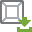.

2. Navigate to the file to import then click Open.

3. The Import Options dialog is displayed.

1. Select the unit of measurement the geometry is measured in.  If you are unsure select the “CAD Units” options and the import will interpret the units used in the CAD file.  It is recommended to specify which units as some CAD formats do not save any units system in the file.

2. If applicable, tick the Merge Sections checkbox to merge separate geometry sections into one single section.

3. Use the Rename using Filename option to name the imported geometries from the CAD file name.

4. Choose a method for the meshing (these options do not affect pre-meshed objects):

1. Rigid Body mesh: Creates mesh to represent the topology of a rigid object.

 Minimum Size Minimum allowable element size. This can be an absolute value or a multiple of the characteristic length. Maximum Size Maximum allowable element size. This can be an absolute value or a multiple of the characteristic length. NB. If there is no limit to the largest element required this can be set to a very large value Maximum Deviation Allowable deviation between the element edge and the surface edge. To meet this requirement, element edge lengths along a curved surface edge are reduced as needed down to a lower limit set by the minimum element size. This can be an absolute value or a multiple of the characteristic length. Maximum Feature Angle Maximum angle across which elements can be maintained. When two adjacent elements’ normals exceed this angle, a new set of nodes is created between them to maintain clean feature lines. Using higher value results in elements spanning the feature line.
2. Size and Bias: Uses elements of a uniform size that you specify to create mesh.

 Element Size Average element size. The length of any active (shared or free) surface edge divided by the element size determines the number of elements to place along that edge.

NB. The parameters for mesh import are based on the units units defined for the main EDEM GUI and are not affected by the unit selected in step 3.
5. Set the geometry material properties by selecting the required geometry and navigating to the general tab.
6. Set the geometry Kinematics as appropriate.

#### Characteristic Length

The Characteristic Length is calculated during the geometry import.  The Characteristic Length is the average length of each feature in the CAD file.  The minimum and maximum length for the Rigid Body meshing can be set as a multiple of the Charateristic Length which will allow the import settings to be related to the specific geometry which is being imported.#### Importing Multiple Geometry Sections Parts

1. Right Click on Geometries in the Creator > Geometry tree and choose Import Geometry.

2. Navigate to the list of files to import and select multiple files either by Ctrl + Left Click and selecting each file or hold down the Shift key and select a list of files.

3. Click open and the import Options dialog is displayed.

1. Select the unit of measurement the geometry is measured in.  Note all selected geometries will be imported with the same units, if geometries have been created with different units they will have to be imported separately.

2. If applicable, tick the Merge Sections checkbox to merge separate geometry sections into one single section. This will merge geometries in the same files together, it won’t merge geometries if they are in separate files. Click OK.

4. Follow the import procedure as per importing a single file.

5. Set the Geometry material properties by selecting the required geometry and navigating to the general tab or select multiple imported geometries to set their material properties.

()

### Repositioning Imported Geometry Sections Parts

Imported CAD geometry sections can be moved (translated) and rotated. You can also export the translation data to a text file.

1. Select the imported section and expand the section tree.

2. Select CAD Geometry then define the transformation as required.

3. Tick the Display Bounding Box checkbox to display a blue box around the geometry section, aligned to its axis.

 Movement Parameters Translation Set the position of a translation vector to determine where to move the geometry to. The direction and magnitude of the translation vector is defined by the start and end points. Once set, specify the distance to move the geometry along the vector the Distance is automatically calculated. Updating the Distance value will update the start and end points.   Pick Start Point. Click to enable the target cross hair, then click a point on a geometry in the Viewer to set the start point for the translation. Pick End Point. Click to enable the target cross hair then click a point on a geometry in the Viewer to set the end point for the translation. Start Point X, Y, Z. Specify the X, Y, and Z coordinates of the start point of the vector along which the geometry is to be translated. End Point X, Y, Z. Specify the X, Y, and Z coordinates of the end point of the vector along which the geometry is to be translated. Distance. Modify the distance to move the geometry along the vector. Display Vector. Click to view the translation vector. Rotation Set the position of a rotation axis to determine how to rotate the geometry. This axis is specified as a vector between two points around which all rotations are performed using a rotation angle. Once set, specify the angle to rotate the geometry around the axis.     Pick Start Point. Click to enable the target cross hair, then click a point on a geometry in the Viewer to set the start point for the rotation. Pick End Point. Click to enable the target cross hair then click a point on a geometry in the Viewer to set the end point for the rotation. Start Point X, Y, Z. Specify the X, Y, and Z coordinates of the start point of the vector along which the geometry is to be rotated. End Point X, Y, Z. Specify the X, Y, and Z coordinates of the end point of the vector along which the geometry is to be rotated. Angle. Specify the angle to rotate the geometry around the vector. Display Axis. Click to view the rotation axis.

Note: when using the Pick Tool for reposition the CAD geometry may ‘jump’ to a new location on selecting the End Point. This is expected behavior as the reposition moves the Geometry along the vector and distance between the selected points.

To export the transformation settings, select File > Export > Geometry Transformations. Choose which sections to export data from, then specify a file. Click the export button to save the transformation data to a text file.

### Geometry Sections Groups

Geometry groups can be used to organize Geometry sections in the creator and analyst trees. Groups can be added by right clicking on the Geometry section of the tree and "Add Geometry Group", or by selecting multiple Geometry sections and then right clicking "Add to new Geometry group" which will create a new group and move the Geometry sections into it. Deleting a group containing Geometry sections will give an option to delete Geometry sections in the group or just the group item itself. If a new Geometry is added/imported while a Geometry section within a group is selected, the new Geometry will be added to this group, otherwise it will be added to the top level Geometry sections group. Copying a Geometry will also now create the copy at the same level in the Geometry tree hierarchy.

Geometry sections can now be moved between or to Geometry groups via a right click context menu option. A Geometry within a Geometry group can be removed from it easily via the right click 'remove from Geometry group' action or the keyboard shortcut ctrl+shift+x.

### Merging Geometry Sections Parts

Geometry sections can be merged at any time.

1. Select all the sections to merge merge holding the ctrl key. The merged sections inherit the section name of the Geometry section first selected.

2. Right click and choose Merge Geometry or use the icon system.

3. Confirm the sections to be merged and select ok.

### Copying Geometry Sections Parts

Created and imported geometry sections can be copied, using the single copy option:

1. Select the geometry section you want to copy.

2. Right click and choose Copy Geometry > Single Copy or use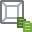.

A copied geometry appears in the same position as the original; it is not offset from the original’s position. The name of the new section will be called New Section N.

Using the multiple copy option:

1. Select the geometry section you want to copy.

2. Right click and choose Copy Geometry > Multiple Copy or use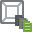.

3. Choose the Number of Copies required and the Default or Original Naming convention.

1. Default naming convention makes copies called “New Section N” where N is between 1 and the number of copies required.

2. Original naming convention makes copies of Geometries keeping the original name. For example Bucket will be copied to Bucket(N) where N is between 0 and ‘number of copies required – 1’.

### Replacing Geometry sections

Geometry sections can be replaced by new imported Geometry objects or the standard EDEM objects.  These replaced objects will maintain the factory, kinematic and transform settings.

1. Select the object to be replaced
2. Right Click and choose “Replace Geometry with”
3. Select a Box, Cylinder, Polygon or CAD Geometry

Note: Geometry sections can only be replaced at time step 0.

### Creating Particle Factories

Particle factories are used to define where, when and how particles/meta-particles appear in a simulation. Any virtual surface or volume (physical or virtual) can be turned into a particle factory Material Generator. Factories can only be created if a Bulk Material has been defined. A simulation can have any number of particle factories.

1. Select the geometry section you want to use as a factory.

2. Right click and choose Add Factory/Add Material Block or use the icon system.

3. Alternatively Select Plugin Factories from the expanded Geometries tab and Right Click to import your own custom particle factory (for details on custom particle factories, refer to the EDEM Programming Guide).

There are two types of particle factory: static and dynamic. To change between factory types use Right Click > Change Factory Type.

#### Static Factories

Static factories produce particles/meta-particle at a specified time. Simulation is paused during particle creation.

 Parameter Details Number or mass of particles For a static factory, select either: Fill Section. Creates as many particles as will fit on or in the chosen geometry section. Total Number. Produce a specified number of particles. Total Mass. Produce a specified amount of mass. Start time Set the time at which particle creation will take place. Max attempts to place particle If you select random particle position, you must also define the maximum number of attempts to place a particle.   During simulation, EDEM attempts to place particles in a random position in or on the specified section. If placing the particle in that position would cause an overlap with any other physical element in the model, the simulator will abandon the position and try to find another. It will attempt this for the specified number of times for each particle. Overlap Check Based on Contact detection between particles can be based on the physical or the contact radius.  If you are generating particles which have a contact radius (for example when using the bonded model) selecting the “Based on Contact Radius” option will ensure that the particles are placed with enough separation to ensure that the particles do not bond to one another.

#### Dynamic Factories

Dynamic factories produce particles over the duration of a simulation. Simulation continues as the particles are created.

 Parameter Details Number or mass of particles For a dynamic factory, select either: Unlimited Number. This creates as many particles as will fit on or in the chosen geometry section. If selected, the factory will attempt to create particles continuously from the specified start time until the end of the simulation. Particles will continue to be created even after a section surface or volume is full. This may cause the rest of the simulation to slow down. Total Number. Produce a specified number of particles. Total Mass. Produce a specified amount of mass. Generation rate Target Number. Define the number of particles the simulator will attempt to place in or on the section every second. Target Mass. Define the mass generation rate the simulator should aim to create. When simulation starts, this rate is converted into mass per time step. If this is less than the smallest particle mass, the factory does not create a particle but adds the mass to the mass for the next time step. Once there is enough mass, the factory creates a particle. If the mass per time step is greater than the smallest particle mass, the factory places particles until it reaches the mass per time step. Be sure your factory is large enough to create the required mass rate: if not, a warning message may be displayed prompting you to update the rate. Note. Target Mass is not compatible with Cubic, BCC and FCC factories. Mass Flow Equation f(t) [kg].  Define a time dependent mass flow equation.  The functions which can be used can be found here.  Note, the units for the mass flow equation is always kilograms. Start time Set the time particle creation generation starts. End time Set the time material generation stops. Max attempts to place particle During simulation, EDEM attempts to place particles in a random position in or on the specified section. If placing the particle in that position would cause an overlap with any other physical element in the model, the simulator will abandon the position and try to find another. It will attempt this for the specified number of times for each particle. Overlap Check Based on Contact detection between particles can be based on the physical or the contact radius.  If you are generating particles which have a contact radius (for example when using the bonded model) selecting the “Based on Contact Radius” option will ensure that the particles are placed with enough separation to ensure that the particles do not bond to one another.

#### Factory Parameters

The parameters area is used to specify the section to use as a factory, and to define the starting conditions of the particles it creates.

 Parameter Options Material/Meta-Particles The Bulk Material created by this factory. Position When particles and meta-particles are created, they are placed on or in the specified section. Particles are never created on top of one another. When a factory tries to create a particle/meta-particle it will repeatedly attempt to find free space to place it.   The position of the particles can be:   Random. Particles are positioned randomly. Cubic. Particles are created in a simple cubic lattice. Select the Display Lattice option in the Viewer controls pane to show the lattice in the Viewer. BCC lattice. Particles are created in a body-centered cubic lattice. It is defined in the same way as a cubic lattice. FCC lattice. Particles are created in a face-centered cubic lattice.  It is defined in the same way as a cubic lattice. Notes: only a random position is selectable when defining a target mass to create. Velocity Particles can be given a velocity at the time of creation. Options are:   Fixed. All particles have the same fixed velocity. The velocity must be defined as a vector.   Fixed. All particles have the same fixed velocity. The velocity must be defined as a vector. Random. Each particle is given a velocity at random within a defined range. Normal distribution. The particle velocities have a normal distribution. The particle direction, mean velocity and the standard deviation must be defined. Linear distribution. The particles are given velocities that fall on the line segment between the two velocity vectors provided by the user. Spray. The particles are formed in a conical spray. The spray direction is defined as a velocity vector. The angle is the angle of maximum deviation from the normal. The mean velocity and standard deviation must also be defined. Orientation Particles can be given an orientation at the time of creation. This is useful for non-spherical particles. The options are as follows:   Fixed. All particles have the same fixed orientation. The orientation is defined in an orientation matrix. EDEM automatically adjusts the values you enter to maintain an orthogonal orientation matrix. Random. Each particle is given a random orientation. Angular velocity Particles can be given an angular velocity at the time of creation i.e. they will be spinning when placed on or in the specified section. The options are as follows:   Fixed. All particles have the same fixed angular velocity. The velocity must be defined as a vector. Random. Each particle is given an angular velocity at random within a defined range. Normal distribution. The particle angular velocities have a normal distribution. The particle direction, average angular velocity and the standard deviation must be defined. Linear distribution. Particles are given angular velocities that fall on an arbitrary arc drawn between two velocity vectors. Custom Property If you have defined any custom properties, particles can be given a custom property value at the time of creation. Options are:   Fixed. All particles have the same fixed value. Initial. Particles use the initial value of the property. Normal. Custom property values have a normal distribution. The mean and standard deviation must be defined. Random. Each particle is given a value at random within a defined range.

#### OpenCL Factory Domain

A domain around the factory is used for OpenCL GPU simulations.  Particles inside the domain use the CPU for the calculation and this can have a significant impact on the simulation performance.  A domain object is drawn for OpenCL GPU factories to indicate the region where the CPU calculations will take place.  Where possible it is advisable to aim to reduce the number of particles in the region to ensure the best performance.

This option allows the user to create a bed of material in the selected geometry. Due to its functionality, the tool is only available for Box geometries. To use select the material box and Right Click to choose Add Block factory. The following Parameters are then shown: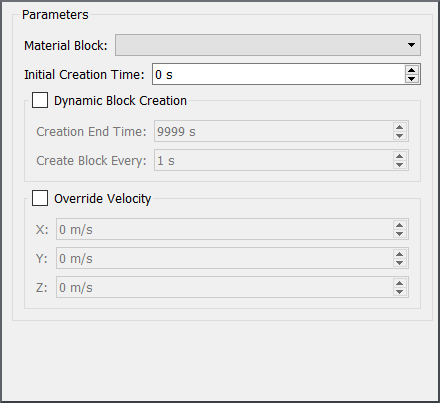Material Block: The dropdown list shows all the saved material blocks within the Material Database that have been transferred to the current simulation deck. Notice that transferring a block from the Material Database to the current simulation also transfers all the relevant Bulk and Equipment materials, interactions and the Contact Model used.

1. Without choosing any additional settings EDEM will place as many copies of the selected block as they fit within the volume of the factory box. This will essentially create a bed of material within the box domain.

2. If Dynamic Block Creation is chosen then EDEM will perform the above action every time period set by the user in the ‘Create Block Every’ section. If the geometry box is the same size as the original saved block then one block will be created every time period, essentially taking the output of one simulation as an input to another.

3. If Override Velocity is chosen EDEM will overwrite the stored material velocity with the user specified value.

EDEM will place as many copies of the selected block as they fit within the volume of the factory box.

### Selecting Geometry Sections Parts

Geometry sections can be selected in the interface by clicking on the required geometry in the viewer window. This then highlights the geometry in the Creator Tree.

Multiple geometries can be selected in the Creator Tree by expanding the Tree and holding down Ctrl while selecting the geometries. The advantage of this method is that once multiple Geometries are selected the Material can be changed or assigned for all selected Geometries via the General > Material option. Alternatively the multiple geometries can be deleted using the Delete key or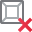.

#### Volume Packing

The Volume packing capability can effectively fill any closed volume to a desired solid fraction.  The volume packing is calculated at the start of a simulation but the creation of the material can be offset to the required start time within the simulation.  To use the volume packing feature:

1. Select a closed volume from the geometries tree
2. Right click “Add volume packing”
3. Define the materials to fill the volume.  This can be a mix of different materials.
4. Select the appropriate Solid fraction (0.7 would be very high, 0.4 very low)

Note: the volume packing tool does not calculate forces based on overlaps, creating material with very high overlaps could lead to a particle explosion once the forces are calculated in the physics model.
5. Set the start time.  This is the time where the particles will enter the simulation.
6. For further configuration of the volume packing tool click on the cog icon.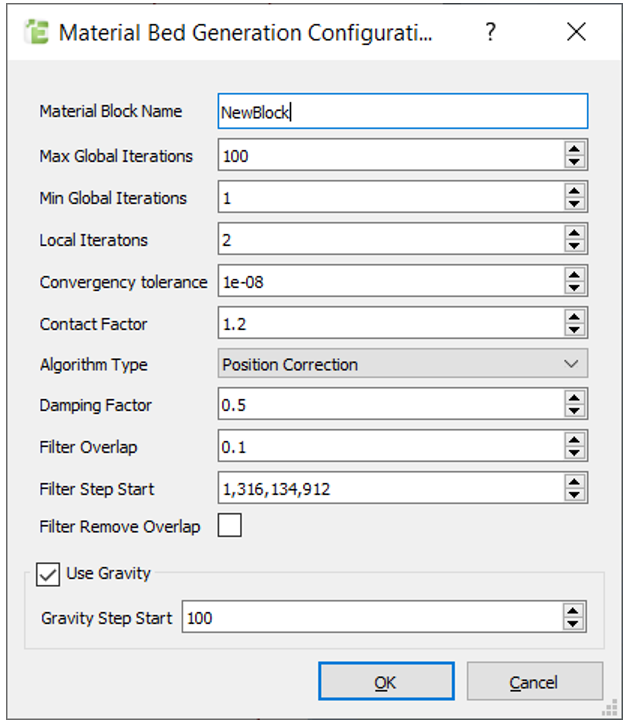Max Global Iterations Maximum number of iterations for the generation Min Global Iterations Minimum number of iterations for the generation Local Iterations Used in the optimization algorithm on each iteration for calculating the new position of particles. Convergency tolerance Used in the optimization algorithm to determine when the bed is fully settled. Contact Factor Contact list is generated from contact factor*physical radius Algorithm Type Position correction algorithm is based on calculating particle displacement based on the average distance to neighbors. optimization algorithm is an algorithm that calculates the displacement by solving an optimization function based on the total distance to particle neighbors and geometry. Damping Factor Value between 0 and 1 used to apply corrections on each iteration. Default value is 0.5 Filter Overlap Criteria to remove particles by overlap. Value representing a fraction of particle’s radius. Filter Step Start Set the start iteration for applying filter. Must be smaller that max number of iterations Filter Remove overlap After exiting the main loop, reduce the particle size to remove any remaining overlap Use Gravity Gravity value taken from creator. Periodic Axes Define the axis which are periodic (see periodic boundaries) to generate material blocks.

#### Filters

Filters are used to remove particles with large overlaps.  The filter is applied after a certain number of iterations and the size of the overlap is user defined.

#### Gravity

As part of the generation process, it is possible to consider the influence of gravity in the system. This influence will result in a deformation of the system based on the material properties of the particles. This deformation is normally achieved during the simulation, allowing the system to find the equilibrium due to gravity forces. This condition requires different steps during the generation process:

1. The system iterates with no gravity condition to allow the system a minimum convergency.
2. The deformation due to gravity is imposed in the system by estimating the position of each particle after deformation.
3. The overlap between particles is modified to emulate the particle force by gravity. This is used to ensure the system is not moving back to original positions during the iterations.